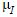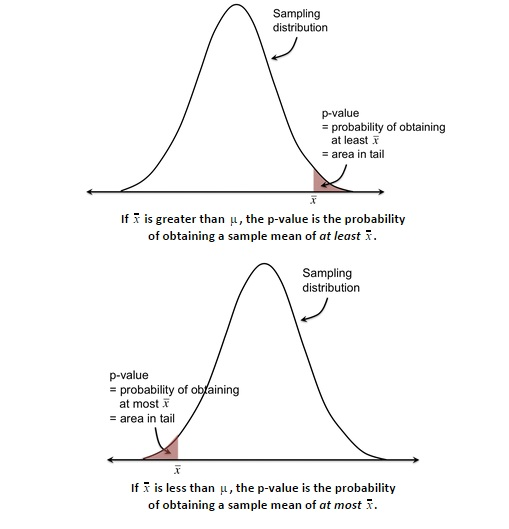PURCHASE A DIGITAL COPY PURCHASE A HARD COPY Lesson 1 Introduction to Statistical Research Methods Lesson 2 Visualizing Data Lesson 3 Central Tendency Lesson 4 Variability Lesson 5 Standardizing Lesson 6 Normal Distribution Lesson 7 Sampling Distributions Lesson 8 Estimation Lesson 9 Hypothesis Testing Lesson 10 t-Tests for Dependent Samples Lesson 11 t-Tests for Independent Samples Lesson 12 Intro to One-Way ANOVA Lesson 13 One-Way ANOVA: Test significance of differences Lesson 14 Correlation Lesson 15 Linear Regression Lesson 16 Chi-Squared Tests Afterward Index

You’ve now seen two ways of judging whether or not a sample mean is likely or unlikely. The first is by looking at where the sample mean falls on the sampling distribution in relation to the population mean. The second is by looking at the confidence interval (typically a 95% CI) for the true population mean if everyone were to have that intervention (), and see if the original population mean falls in this range.

In this lesson, we’ll focus again on the first method&where a sample mean falls on the sampling distribution&and formalize the procedure of deciding whether or not this sample mean is likely or unlikely. We do this by finding the p-value, which is the probability of obtaining that sample mean.Oftentimes, we decide that a sample mean is significantly unlikely if the p-value is less than 0.05 (called our alpha level, or significance level).

To continue learning, purchase Street-Smart Stats: A Friendly Introduction to Statistical Research Methods.